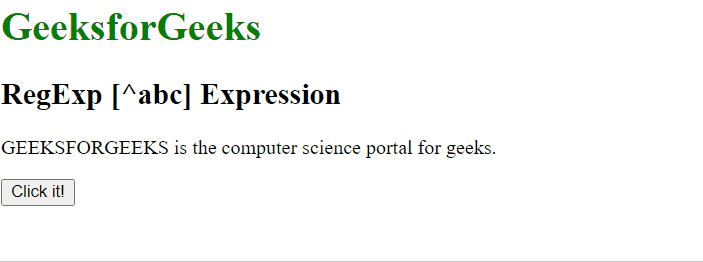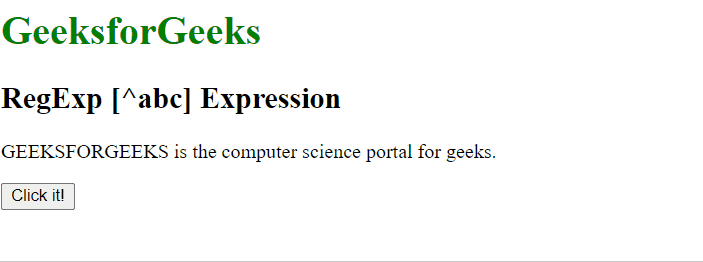GeeksforGeeks App
Open AppBrowser
Continue

# JavaScript RegExp [^abc] Expression

The RegExp [^abc] Expression in JavaScript is used to search for any character which is not between the brackets. The character inside the brackets can be a single character or a span of characters.

• [A-Z]: It is used to match any character from uppercase A to uppercase Z.
• [a-z]: It is used to match any character from lowercase a to lowercase z.
• [A-z]: It is used to match any character from uppercase A to lowercase z.
• [abc…]: It is used to match any character between the brackets.

Syntax:

`/[^abc]/ `

or

`new RegExp("[^abc]")`

Syntax with modifiers:

`/\[^abc]/g `

or

`new RegExp("[^abc]", "g")`

Example 1: This example searches the characters which are not present between [A-G] i.e uppercase A to uppercase G in the whole string.

## html

 ```<``html` `lang``=``"en"``>` `<``head``>``    ``<``title``>``        ``JavaScript RegExp [^abc] Expression``    ````` `<``body``>``    ``<``h1` `style``=``"color:green"``>``        ``GeeksforGeeks``    ``` `    ``<``h2``>RegExp [^abc] Expression` `    ``<``p``>``        ``GEEKSFORGEEKS is the computer``        ``science portal for geeks.``    ``` `    ``<``button` `onclick``=``"geek()"``>``        ``Click it!``    ``` `    ``<``p` `id``=``"app"``>` `    ``<``script``>``        ``function geek() {``            ``let str1 = "GEEKSFORGEEKS is the computer "``                ``+ "science portal for geeks.";` `            ``let regex4 = /[^A-Z]/g;``            ``let match4 = str1.match(regex4);` `            ``document.getElementById("app").innerHTML =``                ``"Found " + match4.length``                ``+ " matches: " + match4;``        ``}``    ```````

Output:RegExp [^abc] Expression

Example 2: This example searches the characters which are not present between [a-g] i.e lowercase a to lowercase g in the whole string.

## html

 ```<``html` `lang``=``"en"``>` `<``head``>``    ``<``title``>``        ``JavaScript RegExp [^abc] Expression``    ````` `<``body``>``    ``<``h1` `style``=``"color:green"``>``        ``GeeksforGeeks``    ``` `    ``<``h2``>RegExp [^abc] Expression` `    ``<``p``>``        ``GEEKSFORGEEKS is the computer``        ``science portal for geeks.``    ``` `    ``<``button` `onclick``=``"geek()"``>``        ``Click it!``    ``` `    ``<``p` `id``=``"app"``>` `    ``<``script``>``        ``function geek() {``            ``let str1 = "GEEKSFORGEEKS is the computer "``                ``+ "science portal for geeks.";` `            ``let regex4 = /[^a-p]/g;``            ``let match4 = str1.match(regex4);` `            ``document.getElementById("app").innerHTML =``                ``"Found " + match4.length``                ``+ " matches: " + match4;``        ``}``    ```````

Output:RegExp [^abc] Expression

Supported Browsers: The browsers supported by RegExp [^abc] Expression are listed below: